# How to get a random sample of data with Power Query

Share

This Power Monday trick is about random sample with Power Query. This is based on my experience of working with large volumes of data.

The other day I have been building a hotel dashboard (more on this later). As part of the dashboard, I wanted to show a random sample of user reviews. Reviews database had quite a few rows, so I wanted to extract a randomized sample of 100 reviews and show them in the report. When you refresh the report (Data > Refresh), then a new set of reviews will be fetched and shown.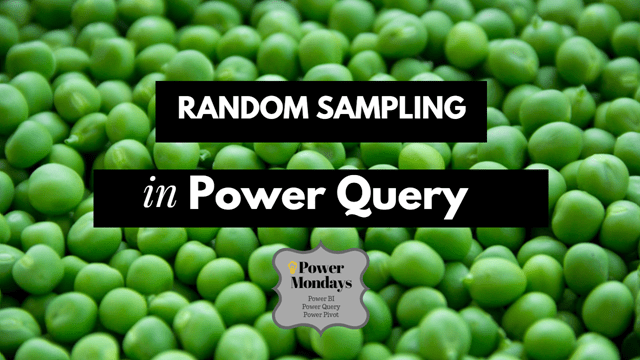Let’s learn how to generate a random sample with Power Query in this article.

This tutorial works in Power Query for Excel or Power BI. In case of Excel, the output sample will be either loaded as table or to data model. In case of Power BI, output goes to your data model.

If you want to get random sample with Excel formulas, read this.

## 5 Steps to create random sample with Power Query

### Step 1: Get your data to Power Query

Simple. Grab the data you want to sample and bring it to PQ. At this point, you will get something like this: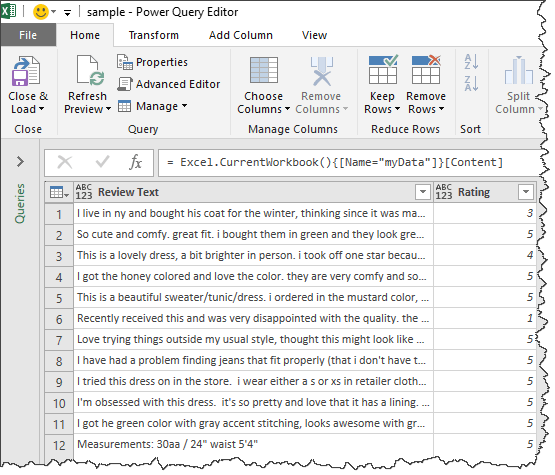### Step 2: Add Random Numbers as a column

=Number.Random()

Remember: Power Query formulas are case-sensitive. So type exactly. Name this column “Random”

### But Power Query gives same random number in all rows …

That is right. As Power Query is a parallel language, each row gets same random number (unlike Excel’s RAND() filled down a column).

Note: your experience with Number.Random() could be different, but as you build transformations, at some point PQ will replace all numbers with same value.

So how to get different numbers per row? Simple, we force PQ to evaluate something per row. A simple thing like index number column will do. This will force PQ to run random formula for all rows.

Hat tip to Gil Raviv for suggesting this technique in a forum post.

### Step 3: Add Index Number column & Sort the random numbers

Go to “Add column” > Index number. Now that we have index numbers in a column, this will force PQ to regenerate the random number per row.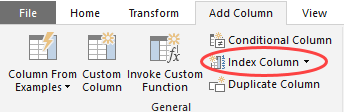Select the random number column and sort it.

### Step 4: Keep top 100 rows

Go to Home > Keep Rows > Keep Top Rows. Enter the sample size you want (100) and Click OK. Your sample is ready.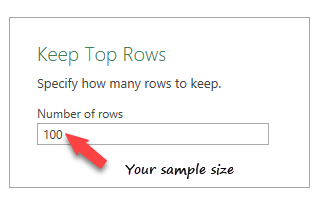### Step 5: Remove the Random & Index columns

Now that our sample is ready, let’s remove the random & index number columns. We do not need them in the final output (or model). Click on Save & Load (or Close & Apply).

Enjoy the sample.

## How to get random sample with repetitions?

The above technique gives a sample without repetitions. What if you need a sample with repetitions (ie memory-less sampling). For example, a series of dice throws or coin tosses?

We can use Power Query to get such samples too. This is slightly complicated compared to first technique, but fun to try.

2. Group the data so you can get row count (while still keeping the data). Like this: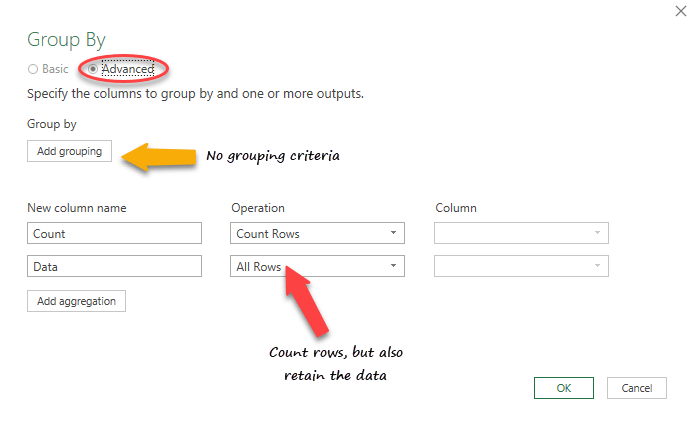3. Add a custom column with a list of 100 numbers =List.Numbers(1,100)
4. Expand the list to new rows
5. Add a column with random number  between 0 & row count-1 =Number.RandomBetween(0,[Count]-1))
7. Change random number to whole number
8. Extract the random row number from [Data] to a new column =[Data]{[Random]}
9. Remove all other columns except this new column in #8
10. Expand the column

Here is the full M code for you to customize.

``````let
Source = Excel.CurrentWorkbook(){[Name="myData"]}[Content],
#"Grouped Rows" = Table.Group(Source, {}, {{"Count", each Table.RowCount(_), type number},
{"Data", each _, type table}}),
#"Expanded List" = Table.ExpandListColumn(#"Added Custom", "List"),
each Number.RandomBetween(0,[Count]-1)),
#"Changed Type" = Table.TransformColumnTypes(#"Added Index",{{"Random", Int64.Type}}),
#"Removed Columns1" = Table.RemoveColumns(#"Removed Columns",{"Count", "List", "Random", "Index"}),
#"Expanded Custom" = Table.ExpandRecordColumn(#"Removed Columns1", "Custom", {"Review Text", "Rating"},
{"Review Text", "Rating"})
in
#"Expanded Custom" ``````

### How to get another sample?

Simple. Just refresh your Power Query connection. You will get another sample.

### How to change the sample size?

In the M code, where it says 100 replace with another number or parameter.

Use Excel Cell to tell Power Query how big a sample you want…

You can even use an Excel named cell to tell PQ what sample size you want. Assuming named cell sample.size has the size, use this M code  =Excel.CurrentWorkbook(){[Name=”sample.size“]}[Content][Column1]{0} to get the value in your query. Use it as part of other steps and bingo, your sample size changes.

### Other questions…?

Excuse the pun, but here is a sample file with all the M code for making your own samples. Examine the queries to learn how this is done.

### How do you sample?

Excel’s Rand() is my favorite way to sample. But now that I am spending more time with Power Query & Power BI, I needed another way to sample the data. This post outlines my preferred approach (unless I am dealing with very large volumes of data) For large volumes of data, I suggest sampling at server-side thru SQL.

New to Power Query? Check out this introduction tutorial.

### Get FREE Excel + Power BI Tips

Simple, fun and useful emails, once per week.

Learn & be awesome.

### Welcome to Chandoo.org

Thank you so much for visiting. My aim is to make you awesome in Excel & Power BI. I do this by sharing videos, tips, examples and downloads on this website. There are more than 1,000 pages with all things Excel, Power BI, Dashboards & VBA here. Go ahead and spend few minutes to be AWESOME.

Read my storyFREE Excel tips book

I will be presenting at two events in London in April 2020. Come & join me.

Chandoo is an awesome teacher
5/5

– Jason

Still on fence about Power BI? In this getting started guide, learn what is Power BI, how to get it and how to create your first report from scratch.

## Related Tips

### 5 Responses to “How to get a random sample of data with Power Query”

1.Chihiro says:

Neat method.

If using PowerBI, you can use Python script to generate random numbers. And then left join original table (using 0 base index) to it.

Sample code for generating 10 random # from 0 to 99.
------------------------------------------------------------
import pandas as pd
import random
df = pd.DataFrame(random.sample(range(100), 10), columns=list('A'))

•Chihiro says:

Ah, figured out something bit more efficient in PowerBI.

In the query editor, go to Transform tab and Run Python Script (note that Preview feature must be turned on).
Run following code.
------------------------------------------
dataset = dataset.sample(len(dataset)//2)
------------------------------------------

This will automatically take approx half of population as sample. Or you can use hard coded number which is smaller than population size. So there is no need for inner join on index.

•Chandoo says:

These tricks are awesome Chihiro. I have not yet tested Python in PBI. I will have a play and may be post a tutorial or two in coming weeks.

2.DragonPhantom says:

When using an [Index] column and a custom "Number.Random()" column I was not able to sort the data, all it would do is recalculate a new random number. So when I was playing around, I found that if add a custom column and add "List.Random(1)" it will give a "List" column and upon expansion it gave me a perfect set of random numbers that don't change and are sort-able! 😀

•Scott says:

THIS was the correct answer. Thank you. The author's steps don't work.

### Get FREE Excel & Power-BI Newsletter

One email per week with Excel and Power BI goodness. Join 100,000+ others and get it free.